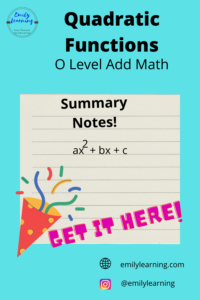Here, you’ll find a summary of what’s tested in the Quadratic function for O Level Additional Mathematics. If you are interested to know what’s in the…## What you need to know for O Level for Quadratic Functions (O Level Add Maths)

In O Level Additional Mathematics, this is what you need to know for quadratic functions: Finding maximum and minimum of a quadratic function by completing…## Adapting to solve this almost linear simultaneous equation for O Level Add Math

Simultaneous equations are often a part of a question in O level Additional Math (also called A Math or Add Maths in short). You can…## Solving Simultaneous Equations – O Level Additional Mathematics

Get our simultaneous equations free course based on the Singapore additional Math syllabus right now! This course consists of 3 video lectures and 1 worksheet…